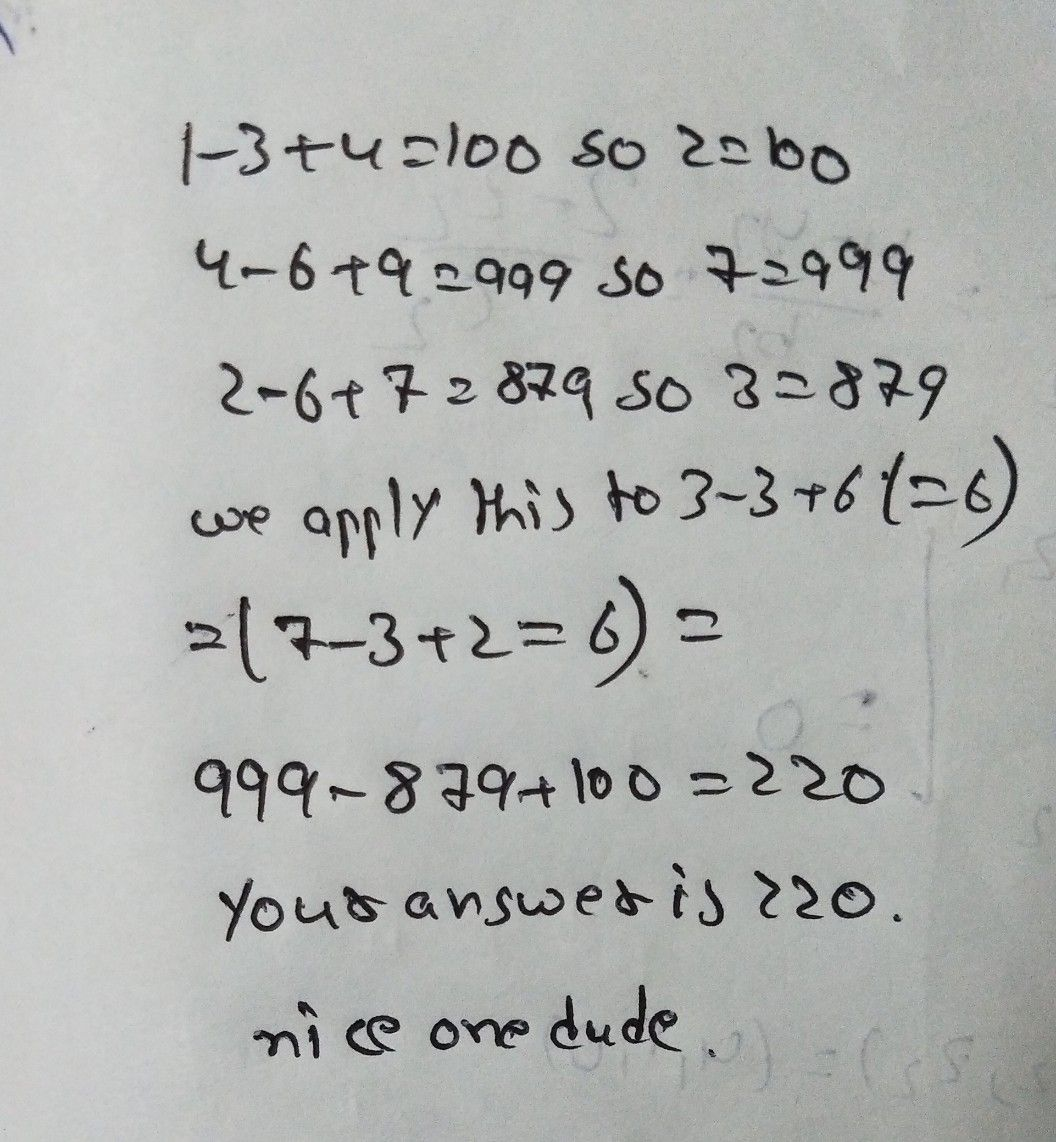Symbol
Problem$1-3+4=100$ $4-6+9=999$ $2-6+7=879$ $3-3+6=73$